# Transportation Problem | Set 6 (MODI Method – UV Method)

There are two phases to solve the transportation problem. In the first phase, the initial basic feasible solution has to be found and the second phase involves optimization of the initial basic feasible solution that was obtained in the first phase. There are three methods for finding an initial basic feasible solution,

This article will discuss how to optimize the initial basic feasible solution through an explained example. Consider the below transportation problem.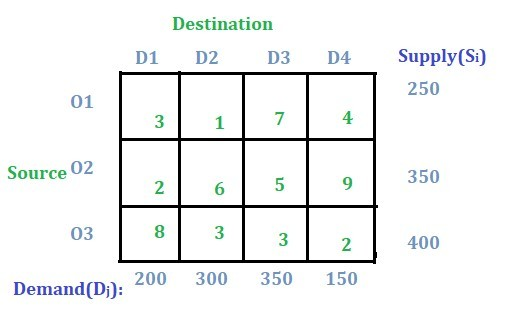Solution:

Step 1: Check whether the problem is balanced or not.
If the total sum of all the supply from sources O1, O2, and O3 is equal to the total sum of all the demands for destinations D1, D2, D3 and D4 then the transportation problem is a balanced transportation problem.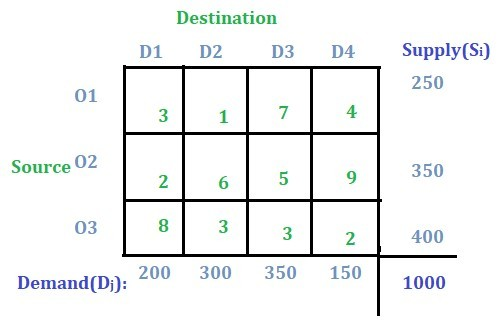Note: If the problem is not unbalanced then the concept of a dummy row or a dummy column to transform the unbalanced problem to balanced can be followed as discussed in this article.

Step 2: Finding the initial basic feasible solution.
Any of the three aforementioned methods can be used to find the initial basic feasible solution. Here, NorthWest Corner Method will be used. And according to the NorthWest Corner Method this is the final initial basic feasible solution: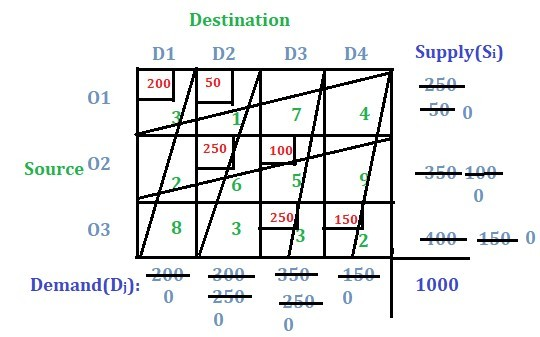Now, the total cost of transportation will be (200 * 3) + (50 * 1) + (250 * 6) + (100 * 5) + (250 * 3) + (150 * 2) = 3700.

Step 3: U-V method to optimize the initial basic feasible solution.
The following is the initial basic feasible solution: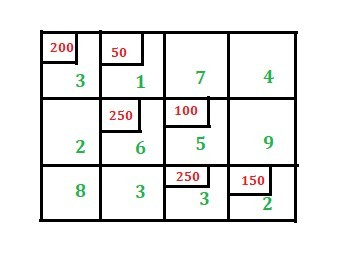– For U-V method the values ui and vj have to be found for the rows and the columns respectively. As there are three rows so three ui values have to be found i.e. u1 for the first row, u2 for the second row and u3 for the third row.
Similarly, for four columns four vj values have to be found i.e. v1, v2, v3 and v4. Check the image below: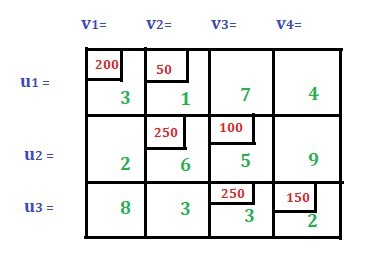There is a separate formula to find ui and vj,
ui + vj = Cij where Cij is the cost value only for the allocated cell. Read more about it here.

Before applying the above formula we need to check whether m + n – 1 is equal to the total number of allocated cells or not where m is the total number of rows and n is the total number of columns.
In this case m = 3, n = 4 and total number of allocated cells is 6 so m + n – 1 = 6. The case when m + n – 1 is not equal to the total number of allocated cells will be discussed in the later posts.

Now to find the value for u and v we assign any of the three u or any of the four v as 0. Let we assign u1 = 0 in this case. Then using the above formula we will get v1 = 3 as u1 + v1 = 3 (i.e. C11) and v2 = 1 as u1 + v2 = 1 (i.e. C12). Similarly, we have got the value for v2 = 1 so we get the value for u2 = 5 which implies v3 = 0. From the value of v3 = 0 we get u3 = 3 which implies v4 = -1. See the image below: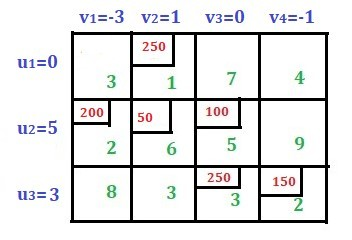Now, compute penalties using the formula Pij = ui + vj – Cij only for unallocated cells. We have two unallocated cells in the first row, two in the second row and two in the third row. Lets compute this one by one.

1. For C13, P13 = 0 + 0 – 7 = -7 (here C13 = 7, u1 = 0 and v3 = 0)
2. For C14, P14 = 0 + (-1) -4 = -5
3. For C21, P21 = 5 + 3 – 2 = 6
4. For C24, P24 = 5 + (-1) – 9 = -5
5. For C31, P31 = 3 + 3 – 8 = -2
6. For C32, P32 = 3 + 1 – 3 = 1

The Rule: If we get all the penalties value as zero or negative values that mean the optimality is reached and this answer is the final answer. But if we get any positive value means we need to proceed with the sum in the next step.

Now find the maximum positive penalty. Here the maximum value is 6 which corresponds to C21 cell. Now this cell is new basic cell. This cell will also be included in the solution.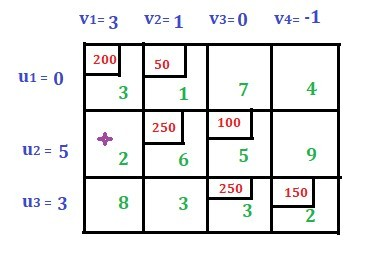The rule for drawing closed-path or loop. Starting from the new basic cell draw a closed-path in such a way that the right angle turn is done only at the allocated cell or at the new basic cell. See the below images: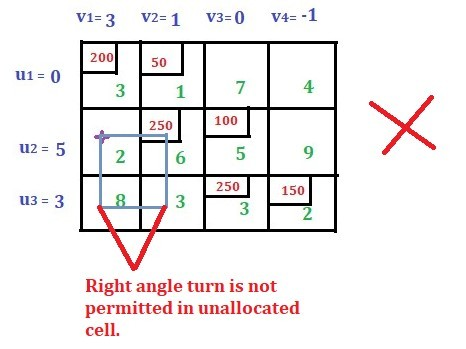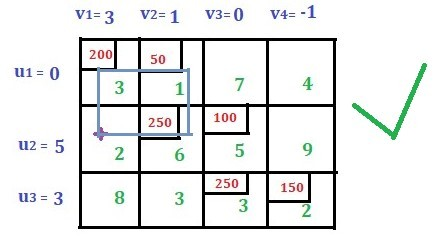Assign alternate plus-minus sign to all the cells with right angle turn (or the corner) in the loop with plus sign assigned at the new basic cell.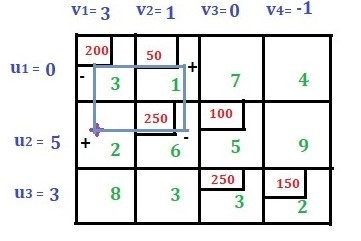Consider the cells with a negative sign. Compare the allocated value (i.e. 200 and 250 in this case) and select the minimum (i.e. select 200 in this case). Now subtract 200 from the cells with a minus sign and add 200 to the cells with a plus sign. And draw a new iteration. The work of the loop is over and the new solution looks as shown below.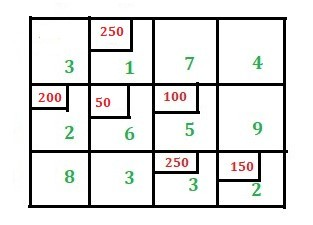Check the total number of allocated cells is equal to (m + n – 1). Again find u values and v values using the formula ui + vj = Cij where Cij is the cost value only for allocated cell. Assign u1 = 0 then we get v2 = 1. Similarly, we will get following values for ui and vj.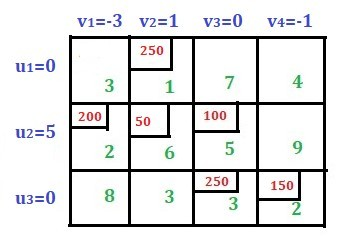Find the penalties for all the unallocated cells using the formula Pij = ui + vj – Cij.

1. For C11, P11 = 0 + (-3) – 3 = -6
2. For C13, P13 = 0 + 0 – 7 = -7
3. For C14, P14 = 0 + (-1) – 4 = -5
4. For C24, P24 = 5 + (-1) – 9 = -5
5. For C31, P31 = 0 + (-3) – 8 = -11
6. For C32, P32 = 3 + 1 – 3 = 1

There is one positive value i.e. 1 for C32. Now this cell becomes new basic cell.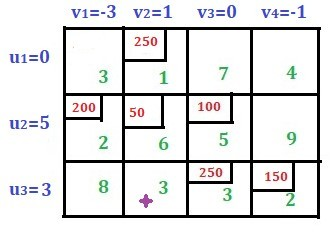Now draw a loop starting from the new basic cell. Assign alternate plus and minus sign with new basic cell assigned as a plus sign.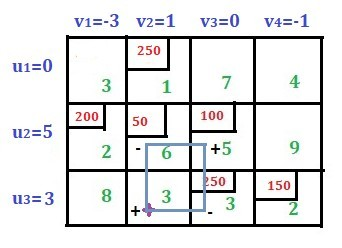Select the minimum value from allocated values to the cell with a minus sign. Subtract this value from the cell with a minus sign and add to the cell with a plus sign. Now the solution looks as shown in the image below: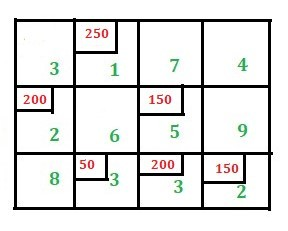Check if the total number of allocated cells is equal to (m + n – 1). Find u and v values as above.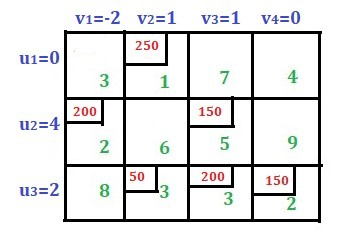Now again find the penalties for the unallocated cells as above.

1. For P11 = 0 + (-2) – 3 = -5
2. For P13 = 0 + 1 – 7 = -6
3. For P14= 0 + 0 – 4 = -4
4. For P22= 4 + 1 – 6 = -1
5. For P24= 4 + 0 – 9 = -5
6. For P31= 2 + (-2) – 8 = -8

All the penalty values are negative values. So the optimality is reached.

Now, find the total cost i.e. (250 * 1) + (200 * 2) + (150 * 5) + (50 * 3) + (200 * 3) + (150 * 2) = 2450

Attention reader! Don’t stop learning now. Get hold of all the important DSA concepts with the DSA Self Paced Course at a student-friendly price and become industry ready.

My Personal Notes arrow_drop_upPossess good Mathematical and Statistical Foundation Data Science Enthusiast Addicted to Python

If you like GeeksforGeeks and would like to contribute, you can also write an article using contribute.geeksforgeeks.org or mail your article to contribute@geeksforgeeks.org. See your article appearing on the GeeksforGeeks main page and help other Geeks.

Please Improve this article if you find anything incorrect by clicking on the "Improve Article" button below.

Improved By : namansnghl

Article Tags :
Practice Tags :

1

Please write to us at contribute@geeksforgeeks.org to report any issue with the above content.